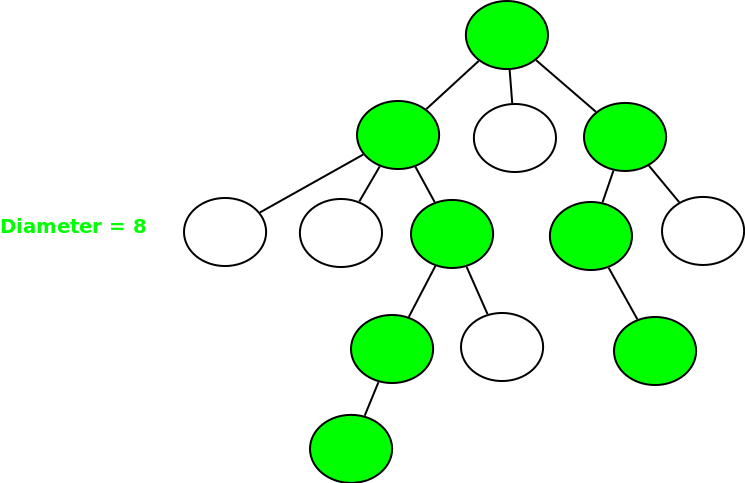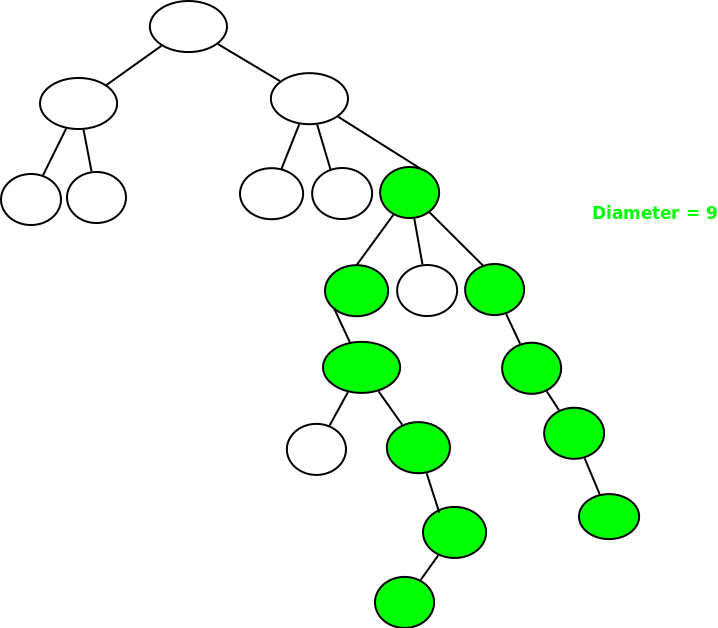Related Articles

# DP on Trees | Set-3 ( Diameter of N-ary Tree )

• Difficulty Level : Medium
• Last Updated : 16 Aug, 2021

Given an N-ary tree T of N nodes, the task is to calculate the longest path between any two nodes(also known as the diameter of the tree).
Example 1:Attention reader! Don’t stop learning now. Get hold of all the important DSA concepts with the DSA Self Paced Course at a student-friendly price and become industry ready.  To complete your preparation from learning a language to DS Algo and many more,  please refer Complete Interview Preparation Course.

In case you wish to attend live classes with experts, please refer DSA Live Classes for Working Professionals and Competitive Programming Live for Students.

Example 2:Different approaches to solve these problems have already been discussed:

In this post, we will be discussing an approach which uses Dynamic Programming on Trees
Prerequisites

There are two possibilities for the diameter to exist:

• Case 1: Suppose the diameter starts from a node and ends at some node in its subtree. Let’s say that there exist a node x such that the longest path starts from node x and goes into its subtree and ends at some node in the subtree itself. Let’s define this path length by dp1[x].
• Case 2: Suppose the diameter or the longest path starts in subtree of a node x, passes through it and ends in it’s subtree. Let’s define this path by dp2[x].

If for all nodes x, we take a maximum of dp1[x], dp2[x], then we will get the diameter of the tree.
For the case-1, to find dp1[node], we need to find the maximum of all dp1[x], where x is the children of node. And dp1[node] will be equal to 1 + max(dp1[children1], dp1[children2], ..)
For the case-2, to find dp2[node], we need to find the two maximum of all dp1[x], where x is the children of node. And dp2[node] will be equal to 1 + max 2 of(dp1[children1], dp1[children2], ..)
We can easily run a DFS and find the maximum of both dp1[node] and dp2[node] for every to get the diameter of the tree.
Below is the implementation of the above approach:

## C++

 `// C++ program to find diameter of a tree``// using DFS.``#include ``using` `namespace` `std;` `int` `diameter = -1;` `// Function to find the diameter of the tree``// using Dynamic Programming``int` `dfs(``int` `node, ``int` `parent, ``int` `dp1[], ``int` `dp2[], list<``int``>* adj)``{` `    ``// Store the first maximum and secondmax``    ``int` `firstmax = -1;``    ``int` `secondmax = -1;` `    ``// Traverse for all children of node``    ``for` `(``auto` `i = adj[node].begin(); i != adj[node].end(); ++i) {``        ``if` `(*i == parent)``            ``continue``;` `        ``// Call DFS function again``        ``dfs(*i, node, dp1, dp2, adj);` `        ``// Find first max``        ``if` `(firstmax == -1) {``            ``firstmax = dp1[*i];``        ``}``        ``else` `if` `(dp1[*i] >= firstmax) ``// Secondmaximum``        ``{``            ``secondmax = firstmax;``            ``firstmax = dp1[*i];``        ``}``        ``else` `if` `(dp1[*i] > secondmax) ``// Find secondmaximum``        ``{``            ``secondmax = dp1[*i];``        ``}``    ``}` `    ``// Base case for every node``    ``dp1[node] = 1;``    ``if` `(firstmax != -1) ``// Add``        ``dp1[node] += firstmax;` `    ``// Find dp``    ``if` `(secondmax != -1)``        ``dp2[node] = 1 + firstmax + secondmax;` `    ``// Return maximum of both``    ``return` `max(dp1[node], dp2[node]);``}` `// Driver Code``int` `main()``{``    ``int` `n = 5;` `    ``/* Constructed tree is``         ``1``        ``/ \``        ``2 3``       ``/ \``       ``4  5 */``    ``list<``int``>* adj = ``new` `list<``int``>[n + 1];` `    ``/*create undirected edges */``    ``adj.push_back(2);``    ``adj.push_back(1);``    ``adj.push_back(3);``    ``adj.push_back(1);``    ``adj.push_back(4);``    ``adj.push_back(2);``    ``adj.push_back(5);``    ``adj.push_back(2);` `    ``int` `dp1[n + 1], dp2[n + 1];``    ``memset``(dp1, 0, ``sizeof` `dp1);``    ``memset``(dp2, 0, ``sizeof` `dp2);` `    ``// Find diameter by calling function``    ``cout << ``"Diameter of the given tree is "``         ``<< dfs(1, 1, dp1, dp2, adj) << endl;` `    ``return` `0;``}`

## Java

 `// Java program to find diameter of a tree using DFS.``import` `java.util.*;``public` `class` `Main``{``    ``// Function to find the diameter of the tree``    ``// using Dynamic Programming``    ``static` `int` `dfs(``int` `node, ``int` `parent, ``int``[] dp1, ``int``[] dp2, Vector> adj)``    ``{``  ` `        ``// Store the first maximum and secondmax``        ``int` `firstmax = -``1``;``        ``int` `secondmax = -``1``;``  ` `        ``// Traverse for all children of node``        ``for` `(``int` `i = ``0``; i < adj.get(node).size(); ++i) {``            ``if` `(adj.get(node).get(i) == parent)``                ``continue``;``  ` `            ``// Call DFS function again``            ``dfs(adj.get(node).get(i), node, dp1, dp2, adj);``  ` `            ``// Find first max``            ``if` `(firstmax == -``1``) {``                ``firstmax = dp1[adj.get(node).get(i)];``            ``}``            ``// Secondmaximum``            ``else` `if` `(dp1[adj.get(node).get(i)] >= firstmax)``            ``{``                ``secondmax = firstmax;``                ``firstmax = dp1[adj.get(node).get(i)];``            ``}``            ``// Find secondmaximum``            ``else` `if` `(dp1[adj.get(node).get(i)] > secondmax)``            ``{``                ``secondmax = dp1[adj.get(node).get(i)];``            ``}``        ``}``  ` `        ``// Base case for every node``        ``dp1[node] = ``1``;``        ``if` `(firstmax != -``1``) ``// Add``            ``dp1[node] += firstmax;``  ` `        ``// Find dp``        ``if` `(secondmax != -``1``)``            ``dp2[node] = ``1` `+ firstmax + secondmax;``  ` `        ``// Return maximum of both``        ``return` `Math.max(dp1[node], dp2[node]);``    ``}``    ` `    ``public` `static` `void` `main(String[] args) {``        ``int` `n = ``5``;``  ` `        ``/* Constructed tree is``             ``1``            ``/ \``            ``2 3``           ``/ \``           ``4  5 */``        ``Vector> adj = ``new` `Vector>();``         ` `        ``for``(``int` `i = ``0``; i < n + ``1``; i++)``        ``{``            ``adj.add(``new` `Vector());``        ``}``      ` `        ``/*create undirected edges */``        ``adj.get(``1``).add(``2``);``        ``adj.get(``2``).add(``1``);``        ``adj.get(``1``).add(``3``);``        ``adj.get(``3``).add(``1``);``        ``adj.get(``2``).add(``4``);``        ``adj.get(``4``).add(``2``);``        ``adj.get(``2``).add(``5``);``        ``adj.get(``5``).add(``2``);``      ` `        ``int``[] dp1 = ``new` `int``[n + ``1``];``        ``int``[] dp2 = ``new` `int``[n + ``1``];``         ` `        ``for``(``int` `i = ``0``; i < n + ``1``; i++)``        ``{``            ``dp1[i] = ``0``;``            ``dp2[i] = ``0``;``        ``}``      ` `        ``// Find diameter by calling function``        ``System.out.println(``"Diameter of the given tree is "``             ``+ dfs(``1``, ``1``, dp1, dp2, adj));``    ``}``}` `// This code is contributed by divyeshrabadiya07.`

## Python3

 `# Python3 program to find diameter``# of a tree using DFS.` `# Function to find the diameter of the``# tree using Dynamic Programming``def` `dfs(node, parent, dp1, dp2, adj):` `    ``# Store the first maximum and secondmax``    ``firstmax, secondmax ``=` `-``1``, ``-``1` `    ``# Traverse for all children of node``    ``for` `i ``in` `adj[node]:``        ``if` `i ``=``=` `parent:``            ``continue` `        ``# Call DFS function again``        ``dfs(i, node, dp1, dp2, adj)` `        ``# Find first max``        ``if` `firstmax ``=``=` `-``1``:``            ``firstmax ``=` `dp1[i]``        ` `        ``elif` `dp1[i] >``=` `firstmax: ``# Secondmaximum``            ``secondmax ``=` `firstmax``            ``firstmax ``=` `dp1[i]``        ` `        ``elif` `dp1[i] > secondmax: ``# Find secondmaximum``            ``secondmax ``=` `dp1[i]` `    ``# Base case for every node``    ``dp1[node] ``=` `1``    ``if` `firstmax !``=` `-``1``: ``# Add``        ``dp1[node] ``+``=` `firstmax` `    ``# Find dp``    ``if` `secondmax !``=` `-``1``:``        ``dp2[node] ``=` `1` `+` `firstmax ``+` `secondmax` `    ``# Return maximum of both``    ``return` `max``(dp1[node], dp2[node])` `# Driver Code``if` `__name__ ``=``=` `"__main__"``:` `    ``n, diameter ``=` `5``, ``-``1` `    ``adj ``=` `[[] ``for` `i ``in` `range``(n ``+` `1``)]``    ` `    ``# create undirected edges``    ``adj[``1``].append(``2``)``    ``adj[``2``].append(``1``)``    ``adj[``1``].append(``3``)``    ``adj[``3``].append(``1``)``    ``adj[``2``].append(``4``)``    ``adj[``4``].append(``2``)``    ``adj[``2``].append(``5``)``    ``adj[``5``].append(``2``)` `    ``dp1 ``=` `[``0``] ``*` `(n ``+` `1``)``    ``dp2 ``=` `[``0``] ``*` `(n ``+` `1``)``    ` `    ``# Find diameter by calling function``    ``print``(``"Diameter of the given tree is"``,``                 ``dfs(``1``, ``1``, dp1, dp2, adj))` `# This code is contributed by Rituraj Jain`

## C#

 `// C# program to find diameter of a tree using DFS.``using` `System;``using` `System.Collections.Generic;``class` `GFG {``   ` `    ``// Function to find the diameter of the tree``    ``// using Dynamic Programming``    ``static` `int` `dfs(``int` `node, ``int` `parent, ``int``[] dp1, ``int``[] dp2, List> adj)``    ``{`` ` `        ``// Store the first maximum and secondmax``        ``int` `firstmax = -1;``        ``int` `secondmax = -1;`` ` `        ``// Traverse for all children of node``        ``for` `(``int` `i = 0; i < adj[node].Count; ++i) {``            ``if` `(adj[node][i] == parent)``                ``continue``;`` ` `            ``// Call DFS function again``            ``dfs(adj[node][i], node, dp1, dp2, adj);`` ` `            ``// Find first max``            ``if` `(firstmax == -1) {``                ``firstmax = dp1[adj[node][i]];``            ``}``            ``// Secondmaximum``            ``else` `if` `(dp1[adj[node][i]] >= firstmax)``            ``{``                ``secondmax = firstmax;``                ``firstmax = dp1[adj[node][i]];``            ``}``            ``// Find secondmaximum``            ``else` `if` `(dp1[adj[node][i]] > secondmax)``            ``{``                ``secondmax = dp1[adj[node][i]];``            ``}``        ``}`` ` `        ``// Base case for every node``        ``dp1[node] = 1;``        ``if` `(firstmax != -1) ``// Add``            ``dp1[node] += firstmax;`` ` `        ``// Find dp``        ``if` `(secondmax != -1)``            ``dp2[node] = 1 + firstmax + secondmax;`` ` `        ``// Return maximum of both``        ``return` `Math.Max(dp1[node], dp2[node]);``    ``}` `  ``static` `void` `Main() {``    ``int` `n = 5;`` ` `    ``/* Constructed tree is``         ``1``        ``/ \``        ``2 3``       ``/ \``       ``4  5 */``    ``List> adj = ``new` `List>();``    ` `    ``for``(``int` `i = 0; i < n + 1; i++)``    ``{``        ``adj.Add(``new` `List<``int``>());``    ``}`` ` `    ``/*create undirected edges */``    ``adj.Add(2);``    ``adj.Add(1);``    ``adj.Add(3);``    ``adj.Add(1);``    ``adj.Add(4);``    ``adj.Add(2);``    ``adj.Add(5);``    ``adj.Add(2);`` ` `    ``int``[] dp1 = ``new` `int``[n + 1];``    ``int``[] dp2 = ``new` `int``[n + 1];``    ` `    ``for``(``int` `i = 0; i < n + 1; i++)``    ``{``        ``dp1[i] = 0;``        ``dp2[i] = 0;``    ``}`` ` `    ``// Find diameter by calling function``    ``Console.WriteLine(``"Diameter of the given tree is "``         ``+ dfs(1, 1, dp1, dp2, adj));``  ``}``}` `// This code is contributed by decode2207.`

## Javascript

 ``
Output:
`Diameter of the given tree is 4`

My Personal Notes arrow_drop_up